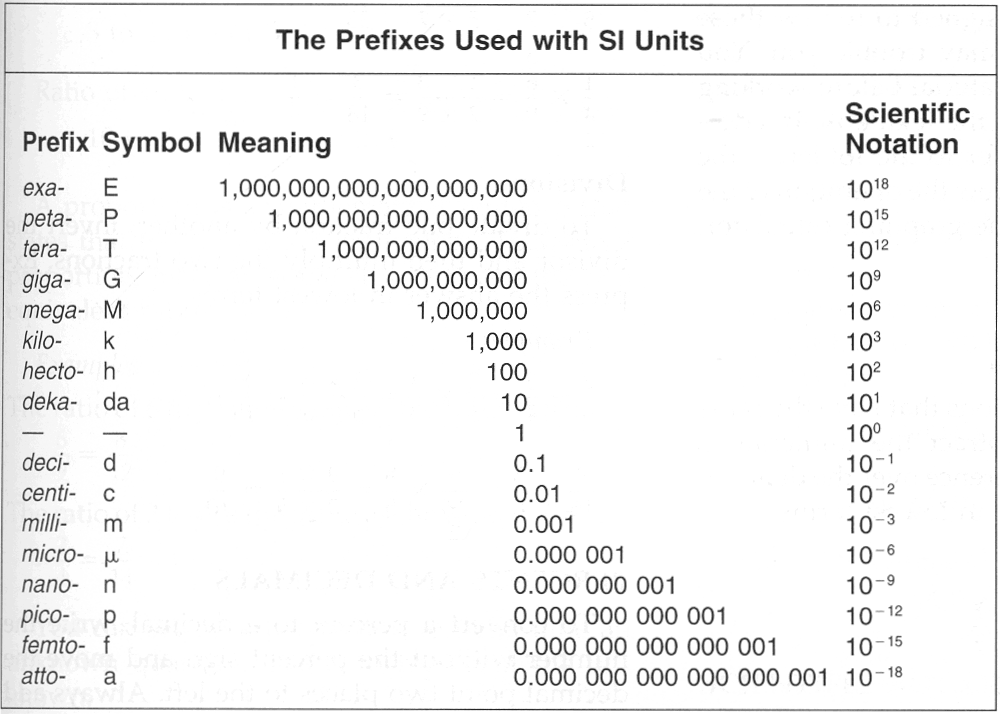Observer:
Presenter:
Recorder:
Date:

## Group Activity: SI Units Conversions 1

### Introduction

Next SI Conversions Activity

The central issue in converting units in the SI system is to figure out how many small units equal one big unit. Here is how to do it:

Take a conversion from millimeters to kilometers. Look at the power of ten next to kilo- in the chart: 103. Now look at the power of ten next to milli- in the chart: 10-3. Divide the power of ten next to the larger unit by the power of ten next to the smaller unit:

``` 103
———— = 106 (when dividing numbers raised
10-3        to an exponent, subtract exponents)
1 x 106 mm
1 x 106 mm = 1 km or  ————————
1 km
```

This result says that there are 106 (1,000,000) millimeters in 1 kilometer. Try another one: how many nanometers (the smaller unit) are there in 1 centimeter (the larger unit)? Nano- is 10-9 and centi- is 10-2.

``` 10-2
———— = 107 or 10,000,000   So there are 1 x 107 (10,000,000) nm in 1 cm.
10-9
```Examples:
```How many mL are there in one L?
base    100
————— = 103  which means 1 L = 1,000 mL
milli-  10-3
How many pg are in one μg?
μ-      10-6
————— = 106  which means 1 μg = 1,000,000 pg
pico-   10-12
Now convert 5,897,000 pg to μg:
1 μg
5,897,000 pg × —————————— = 5.897 μg
1,000,000 pg
```

page break

1. How many pico-units are in one nano-unit?
2. How many pico-units are in one milli-unit?
3. How many nanometers are in one meter?
4. How many milliliters are in one kiloliter?
5. How many centimeters are in one megameter?
6. How many decimeters are in one kilometer?
7. How many femtometers are in one nanometer?
8. How many kilobytes are in one gigabyte?
1. Convert 54 nL to pL
2. Convert 42,000,000 pL to mL
3. Convert 4,009,450,000 nm to m
4. Convert 12 kL to mL
5. Convert 14 Mm to cm
6. Convert 4 km to dm
7. Convert 12 nm to fm
8. Convert 42,000,000 kB to GB
Last updated: Sep 02, 2006        Keller Home  |